# Permutation

Jump to: navigation, search

ofelements

A finite sequence of lengthin which all the elements are different, i.e. a permutation is an arrangement ofelements without repetition. The number of permutations is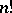.

Usually, one takes as the elements to be permuted the elements of the set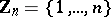; a one-to-one mappingofonto itself defines the permutation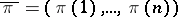. The mappingis also called a substitution of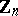. Many problems related to the enumeration of permutations are formulated in terms of substitutions, such as, for example, the enumeration of permutations with various restrictions on the positions of the permuted elements (cf. e.g. , ). A permutation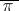can be regarded as an ordered set consisting ofelements if one assumes that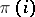precedes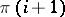,.

Examples. 1) The pair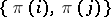forms an inversion inif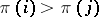for; if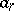is the number of permutations ofelements withinversions, then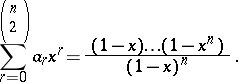2) If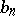is the number of permutationsconsisting ofelements such thatforeven andforodd, then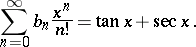Often a permutation is defined to be a bijective mapping of a finite set onto itself, i.e. a substitution (cf. also Permutation of a set).